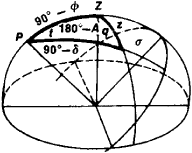# Parallactic Triangle

The following article is from The Great Soviet Encyclopedia (1979). It might be outdated or ideologically biased.

## Parallactic Triangle

in astronomy, the spherical triangle on the celestial sphere with vertices at the celestial pole P, the zenith Z of the observation site, and a given point cr on the celestial sphere; in most cases, σ is the center of a celestialFigure 1

body (see Figure 1). The sides of the triangle are equal to z, 90° — δ, and 90° — Φ, where z and δ are, respectively, the zenith distance and declination of σ and Φ is the latitude of the observation site. Two of the angles are equal to t and 360° — A, where t and A are, respectively, the hour angle and the azimuth, measured from the north point, of σ; the third angle is called the parallactic angle and is denoted by q. By applying the formulas of spherical trigonometry to the parallactic triangle, we can find the equatorial coordinates t and δ of the point σ from the point’s horizontal coordinates A and z, and vice versa:

cos z = sin Φ sin δ + cos Φ cos δ cos t

sin z cos A = -cos Φ sin δ + sin Φ cos δ cos t

sin z sin A = cos δ sin t

The parallactic triangle can also be used to determine the times and azimuths of the risings and settings of celestial bodies (in this case z = 90°) and to compute the time of onset of twilight.